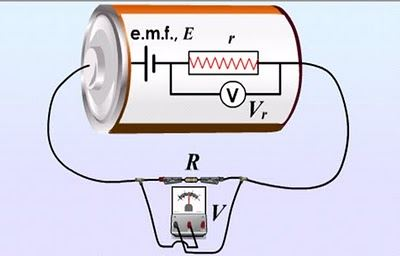# Current Electricity

A few minutes of power outage makes us realize how important electric current is to us. Many inventions and discoveries have been made in order to facilitate human life smoothly. The discovery of current electricity is one such discovery that we are highly dependent on to make our life easier. Here, in this article let us learn about current electricity and its characteristics.

## What is Current Electricity?

Any motion of charges from one section to another is current. When two bodies at different potentials are linked with a wire, free electrons stream from B to A, until both the objects reach the same potential, after which the current stops flowing. Until a potential difference is present throughout a conductor, current runs. The division of physics that deals with charges in motion is termed as current electricity.

### Electromotive Force (EMF) and Voltage:Just as a liquid in a horizontal tube does not flow, no current runs in a copper cable by itself. Water will flow out of the other end at a steady rate if one end of the tube is attached to a tank with water such that there is a pressure difference amidst the two ends of the horizontal tube.

## Resistance and Resistivity

If the physical conditions i.e. temperature, mechanical strain, etc. remain constant It was discovered through experimentation that the current traveling through a conductor is directly proportional to the potential difference V in between its two ends.

### Origin of Resistivity

Inside the conductor, the electric charge is nil everywhere, and thus, there is no current in electrostatic situations. This does not necessarily convey that all charges inside the conductor are at rest.

### Temperature Dependence of Resistivity

The resistivity of a metallic conductor virtually at all times grows with increasing temperature.

## Confines of Ohm’s Law

There are many universally used circuit elements that do not obey Ohm’s law as it is not a fundamental law of nature.

### Superconductivity

Some substances, including numerous alloys and metals, display a phenomenon termed as superconductivity.

### Resistors in Series and in Parallel:

When resistors, can be linked in such a way that equal amounts of current flow in them, then they are said to be attached in series. The resistors are believed to be linked in parallel if the electric potential difference is similar across each resistor.

## Electric Circuits and Kirchhoff’s Rules

Ohm’s law cannot be applied to many electrical circuits. This is caused when resistors are linked in a complex manner or when there is more than one source of emf in the circuit. Two laws founded on charge neutrality in metal were developed by Gustav Robert Kirchhoff to solve such complicated circuits.

## Summary:

• Current flowing through an agreed area of a conductor is the net charge traveling per unit time through the area.
• The division of physics that deals with charges in motion is termed as current electricity.
• The resistivity of a metallic conductor virtually at all times grows with increasing temperature.
• When resistors, can be linked in such a way that equal amounts of current flow in them, then they are said to be attached in series.
• The resistors are believed to be linked in parallel if the electric potential difference is similar across each resistor.### The internal resistance of a cell is the same as the resistance of which factor?

The internal resistance of a cell is the same as the resistance of an electrolyte because the electrolyte is responsible for the conduction of electricity inside the cell.

### On what basis are Kirchhoff’s first and second laws based on?

Kirchhoff’s first law is based on conservation of charge while Kirchhoff’s second law is based on the conservation of energy.

### What will be the resistance of a bundle of a wire which is cut into 4 pieces? The actual resistance of the wire is R.

When the actual wire is cut into 4 pieces, the length of the wire reduces by 1/4. The area will increase by 4 times as the actual wire is cut into 4 pieces. Using the resistance formula, $R=\rho \frac{l}{a}$. Therefore, the new resistance of the bundle of pieces will be R/16.

### What happens to the conductance, if the length of the wire is doubled?

When the length of the wire is doubled, the resistance of the wire will increase by two times. We know that conductance is the reciprocal of resistance. Therefore, conductance will be half.

### What happens to the spring, if the current is passed through a spring?

The spring will compress because of the current flow through the adjacent turns. When the current flows through two wires, they exert an attractive force towards each other resulting in compression of the wire.